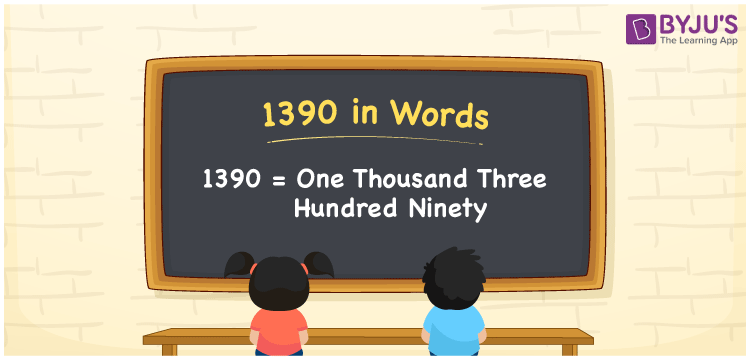# 1390 in Words

1390 in words is given by One Thousand Three Hundred Ninety. Khushi purchased a bicycle worth Rs.1390, then you can say, “Khushi purchased a bicycle worth Rupees One Thousand Three Hundred Ninety”. The number 1390 is a cardinal number as it denotes a specific amount. This article provides information on how to write the number name for 1390 with the help of a place value chart. Also, learn how to spell the number 1390 in English words.

 1390 in Words One Thousand Three Hundred Ninety One Thousand Three Hundred Ninety in numerical form 1390

## 1390 in English Words

Generally, we write numbers in words with the help of the English alphabet for better understanding of numbers in word form. Therefore, the number 1390 in English can be spelt as One Thousand Three Hundred Ninety.## How to Write 1390 in Words?

Let us make a place value chart of 4 columns as the number 1390 is a four-digit number. The below table represents the place value chart for 1390.

 Thousands Hundreds Tens Ones 1 3 9 0

Hence, we can write the expanded form as:

1 x Thousand + 3 x Hundred + 9 x Ten + 0 x One

= 1 x 1000 + 3 x 100 + 9 x 10 + 0 x 1

= 1000 + 300 + 90 + 0

= 1000 + 300 + 90

= 1390

= One Thousand Three Hundred Ninety

Therefore, 1390 in words is written as One Thousand Three Hundred Ninety

Interesting way of writing 1390 in words

1 = One

13 = Thirteen

139 = One Hundred and Thirty-Nine

1390 = One Thousand Three Hundred Ninety

Thus, the word form of the number 1390 is One Thousand Three Hundred Ninety

1390 is a natural number that is one less than 1391 and one greater than 1389

• 1390 in words – One Thousand Three Hundred Ninety
• Is 1390 an odd number? – No
• Is 1390 an even number? – Yes
• Is 1390 a perfect square number? – No
• Is 1390 a perfect cube number? – No
• Is 1390 a prime number? – No
• Is 1390 a composite number? – Yes

## Frequently Asked Questions on 1390 in Words

Q1

### Write 1390 in English words.

1390 in English words is written as One Thousand Three Hundred Ninety.
Q2

### What is the value of 1000 + 390 in words?

1000 + 390 = 1390. Thus the number 1390 in words is One Thousand Three Hundred Ninety.
Q3

### What is the place value of 1 in 1390?

The place value of 1 in 1390 is Thousands.